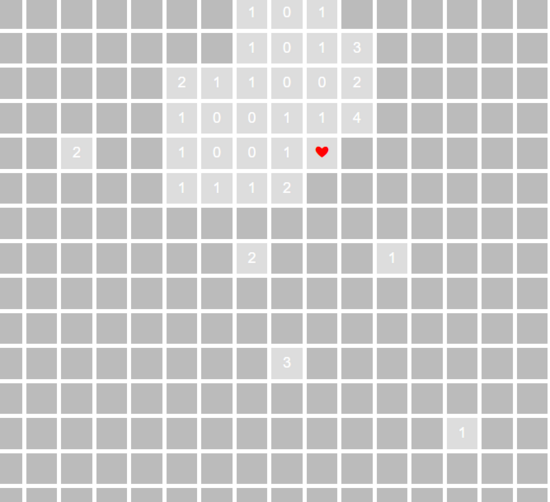# 阿望教你用vue写扫雷小游戏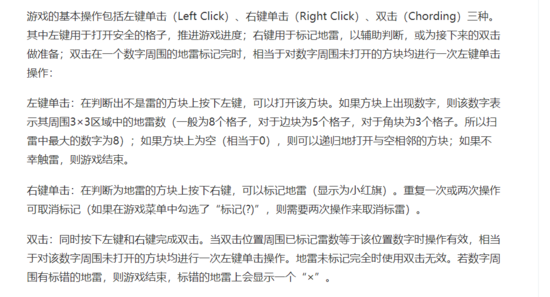1. 难度选择
2. 根据选择的游戏难度绘制游戏主界面，就是格盘
3. 根据选择的游戏难度随机生成地雷放到盘中
4. 计算每个格子周围的雷数
5. 完成用户交互（左键点击翻格子，右键点击放雷）
6. 空白格扩散翻格子
7. 计算游戏结束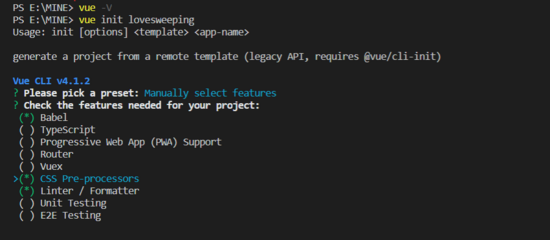``````
- src
- components
- SelectLevel.vue [新增，难度选择组件]
- LoveSweeping.vue [新增，游戏界面组件]
- App.vue [父组件，负责组件间的切换和某些数据传递]
- main.js
- package.json``````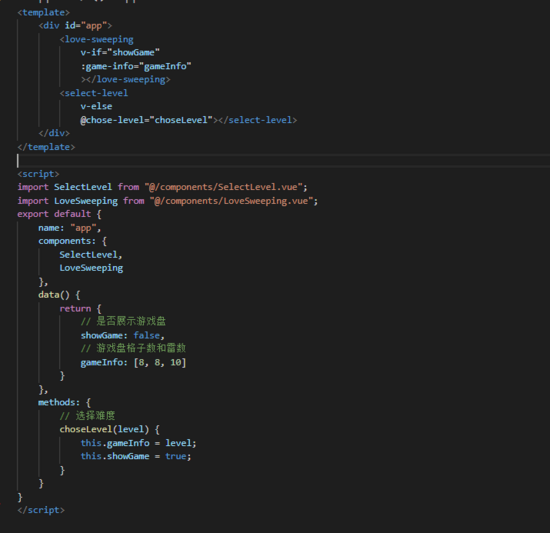``````
// 难度
level: [
{
text: '青铜', // 难度描述
value: [9, 9, 10] // 格子横排数，格子纵排数，雷数
}, {
text: '黄金',
value: [12, 9, 20]
]``````

``````
<li v-for="(item, index) in level" :key="index" @click="handleChoseLevel(item.value)"></li>``````

``````
// 选择难度
handleChoseLevel(level) {
this.\$emit("chose-level", level);
}``````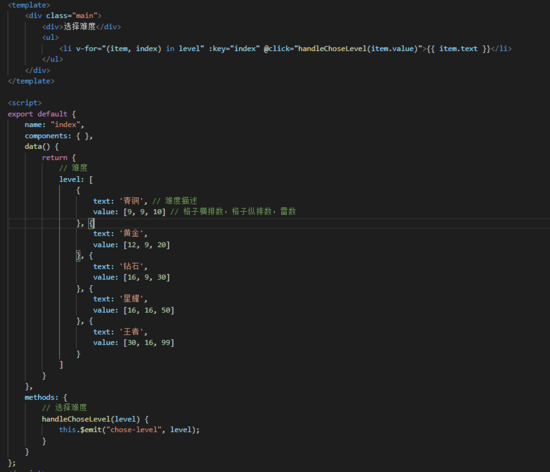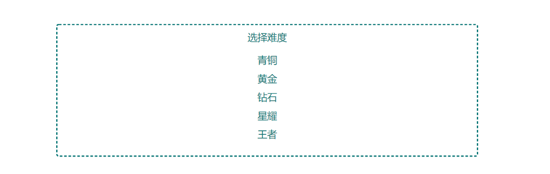``````
<div
v-for="col in cols" :key="Math.random() + col"
class="game-content-row">
<span
v-for="row in rows" :key="Math.random() + row"
class="game-block">
<span></span>
</span>
</div>``````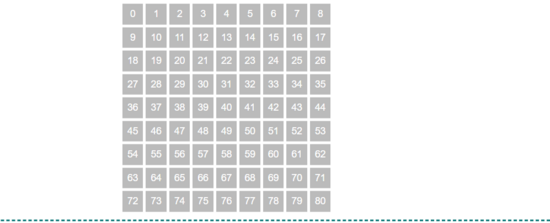``````
// 随机获取雷点位置
getMinePosition() {
// 定义一个数组装不重复的格点
let mineArr = [];
// 循环雷数生成不重复的雷点
for (let n = 0; n < this.gameInfo; n++) {
const random = Math.floor(Math.random() * this.latticeNum);
if (mineArr.indexOf(random) === -1) {
mineArr.push(random);
} else {
n--;
}
}
this.minePosition = mineArr;
},````````````
// 格子属性
lattice: [{
index: 0, // 格子索引
mineNum: 0, // 周围雷数
isMine: false, // 是否是雷
isOpen: false, // 是否已经被点开
isMark: false, // 是否被标记
}],``````

``````
// 获取格子周围的雷数，
getMineNumAroundLattice(lattice, index) {
// 先获取格子周围的有效索引
const latticeIndexArr = this.getLatticeIndex(index);
// 循环索引，索引值在雷点数组中的，即为雷，当前格子的雷点数加1
latticeIndexArr.forEach(i => {
if (this.minePosition.indexOf(i) > -1) {
lattice.mineNum ++;
}
});
},
// 获取格子周围的有效索引
getLatticeIndex(index) {
// 存索引值的变量
let latticeIndexArr = [];
// 当前格子位于第几行
const latticeRow = Math.ceil(index / this.rows);
// 当前格子位于第几列（求余为0说明是最右边一列）
const latticeCol = Math.ceil(index % this.rows) || this.rows;
// 第一行没有上一行，不需要计算减1的行值，最后一行没有下一行，不需要计算加1的行值
for (let i = (latticeRow === 1 ? 0 : -1); i < (latticeRow === this.cols ? 1 : 2); i++) {
// 第一列没有左列，不需要计算减1的列值，最后一列没有右列，不需要计算加1的列值
for (let j = (latticeCol === 1 ? 0 : -1); j < (latticeCol === this.rows ? 1 : 2); j++) {
// 索引值 = (当前行值 + （上一行【-1】/当前行【0】/下一行【+1】） - 1【1是索引从0开始，所以需要减去】) * 每行格子数 + 当前列值 + （上一列【-1】/当前列【0】/下一列【+1】）
const latticeIndex = (latticeRow + i - 1) * this.rows + (latticeCol + j);
latticeIndexArr.push(latticeIndex);
}
}
return latticeIndexArr;
},``````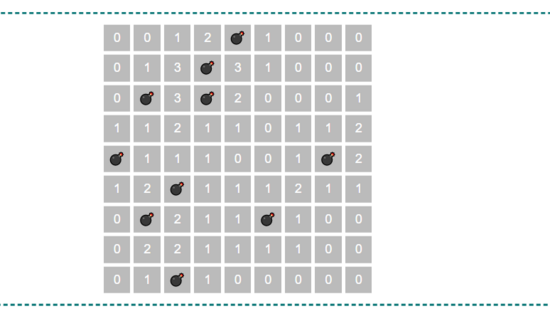``````
@click.left="handleClickLattice(lattice[(col - 1) * rows + row - 1])"
// 点了格子
handleClickLattice(lattice) {
// 是数字
if (lattice.mineNum) {
if (!lattice.isOpen && !lattice.isMark) {
lattice.isOpen = true;
}
}
},``````

``````
// 代码把下半部分补齐
handleClickLattice(lattice) {
... else {
// 是空白
const latticeIndexArr = this.getLatticeIndex(lattice.index);
this.showWhiteAround(lattice, latticeIndexArr);
}
},
// 展示周围的空白标记，直至边缘（格子边缘或者数字）
showWhiteAround(lattice, latticeIndexArr) {
// 避免有重复的数据停不下来，去个重
latticeIndexArr = [...new Set(latticeIndexArr)];
for (let i = 0; i < latticeIndexArr.length; i++) {
const item = latticeIndexArr[i];
latticeIndexArr.splice(i, 1);
i--;
if (this.lattice[item].isOpen) {
continue;
}
this.lattice[item].isOpen = true;
if (!this.lattice[item].mineNum) {
const arr = this.getLatticeIndex(this.lattice[item].index);
this.showWhiteAround(this.lattice[item], latticeIndexArr.concat(arr));
}
}
},``````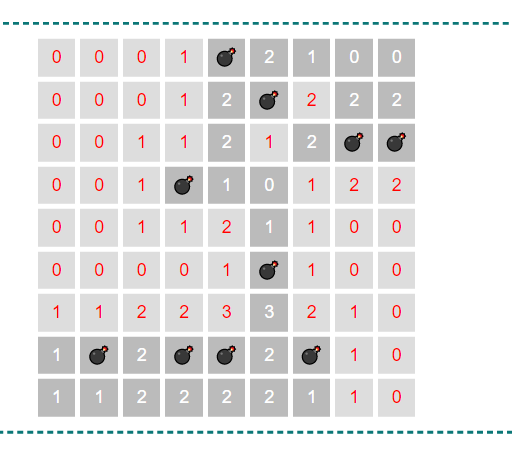``````
// 右键确认是雷点
handleSureMinePoint(lattice) {
if (!lattice.isOpen) {
lattice.isMark = true;
lattice.isOpen = true;
this.minePosition.splice(this.minePosition.indexOf(lattice.index), 1);
this.judgeIsOver();
} else {
if (lattice.isMark) {
lattice.isMark = false;
lattice.isOpen = false;
this.minePosition.push(lattice.index);
}
}
},``````